# How to Calculate and Solve for Pressure Gradient | Polymer & Textile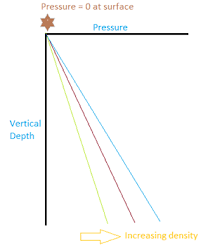The image above represents pressure gradient.

To compute for pressure gradient, four essential parameters are needed and these parameters are value (α), viscosity (μ), screw rotation speed (N) and proportionality constant that depends on screw geometry (B).

The formula for calculating pressure gradient:

ΔP = αμN / B

Where;

α = Value
μ = Viscosity
N = Screw Rotation Speed
B = Proportionality Constant that Depends on Screw Geometry

Let’s solve an example;
Find the pressure gradient when the value is 2, viscosity is 9, screw rotation speed is 20 and proportionality constant that depends on screw geometry is 24.

This implies that;

α = Value = 2
μ = Viscosity = 9
N = Screw Rotation Speed = 20
B = Proportionality Constant that Depends on Screw Geometry = 24

ΔP = αμN / B
ΔP = (2)(9)(20) / 24
ΔP = 360 / 24
ΔP = 15

Therefore, the pressure gradient is 15.

Calculating the Value when the Pressure Gradient, Viscosity, Screw Rotation Speed and Proportionality Constant that Depends on Screw Geometry is Given.

α = ΔP x B / μN

Where;

α = Value
μ = Viscosity
N = Screw Rotation Speed
B = Proportionality Constant that Depends on Screw Geometry

Let’s solve an example;
Find the value when the pressure gradient is 20, viscosity is 5, screw rotation speed is 11 and the proportionality constant that depends on screw geometry is 7.

This implies that;

ΔP = Pressure Gradient = 20
μ = Viscosity = 5
N = Screw Rotation Speed = 11
B = Proportionality Constant that Depends on Screw Geometry = 7

α = ΔP x B / μN
α = 20 x 7 / (5)(11)
α = 140 / 55
α = 2.54

Therefore, the value is 2.54.

Calculating the Viscosity when the Pressure Gradient, Value, Screw Rotation Speed and Proportionality Constant that Depends on Screw Geometry is Given.

μ = ΔP x B / αN

Where;

μ = Viscosity
α = Value
N = Screw Rotation Speed
B = Proportionality Constant that Depends on Screw Geometry

Let’s solve an example;
Find the viscosity when the pressure gradient is 34, the value is 10, the screw rotation speed is 12 and the proportionality constant that depends on screw geometry is 3.

This implies that;

ΔP = Pressure Gradient = 34
α = Value = 10
N = Screw Rotation Speed = 12
B = Proportionality Constant that Depends on Screw Geometry = 3

μ = ΔP x B / αN
μ = 34 x 3 / (10)(12)
μ = 102 / 120
μ = 0.85

Therefore, the viscosity is 0.85.

Calculating the Screw Rotation Speed when the Pressure Gradient, Value, Viscosity and Proportionality Constant that Depends on Screw Geometry is Given.

N = ΔP x B / αμ

Where;

N = Screw Rotation Speed
α = Value
μ = Viscosity
B = Proportionality Constant that Depends on Screw Geometry

Let’s solve an example;
Find the screw rotation speed when the pressure gradient is 48, value is 12, viscosity is 8 and proportionality constant that depends on screw geometry is 9.

This implies that;

ΔP = Pressure Gradient = 48
α = Value = 12
μ = Viscosity = 8
B = Proportionality Constant that Depends on Screw Geometry = 9

N = ΔP x B / αμ
N = 48 x 9 / (12)(8)
N = 432 / 96
N = 4.5

Therefore, the screw rotation speed is 4.5.

Calculating the Proportionality Constant that Depends on Screw Geometry when the Pressure Gradient, Value, Viscosity and Screw Rotation Speed is Given.

B = αμN / ΔP

Where;

B = Proportionality Constant that Depends on Screw Geometry
α = Value
μ = Viscosity
N = Screw Rotation Speed

Let’s solve an example;
Find the proportionality constant that depends on screw geometry when the value is 4, pressure gradient is 12, viscosity is 7 and screw rotation speed is 8.

This implies that;

ΔP = Pressure Gradient = 12
α = Value = 4
μ = Viscosity = 7
N = Screw Rotation Speed = 8

B = αμN / ΔP
B = (4)(7)(8) / 12
B = 224 / 12
B = 18.67

Therefore, the proportionality constant that depends on screw geometry is 18.67.

Nickzom Calculator – The Calculator Encyclopedia is capable of calculating the pressure gradient.

To get the answer and workings of the pressure gradient using the Nickzom Calculator – The Calculator Encyclopedia. First, you need to obtain the app.

You can get this app via any of these means:

To get access to the professional version via web, you need to register and subscribe for NGN 1,500 per annum to have utter access to all functionalities.
You can also try the demo version via https://www.nickzom.org/calculator

Apple (Paid) – https://itunes.apple.com/us/app/nickzom-calculator/id1331162702?mt=8
Once, you have obtained the calculator encyclopedia app, proceed to the Calculator Map, then click on Polymer & Textile under Engineering.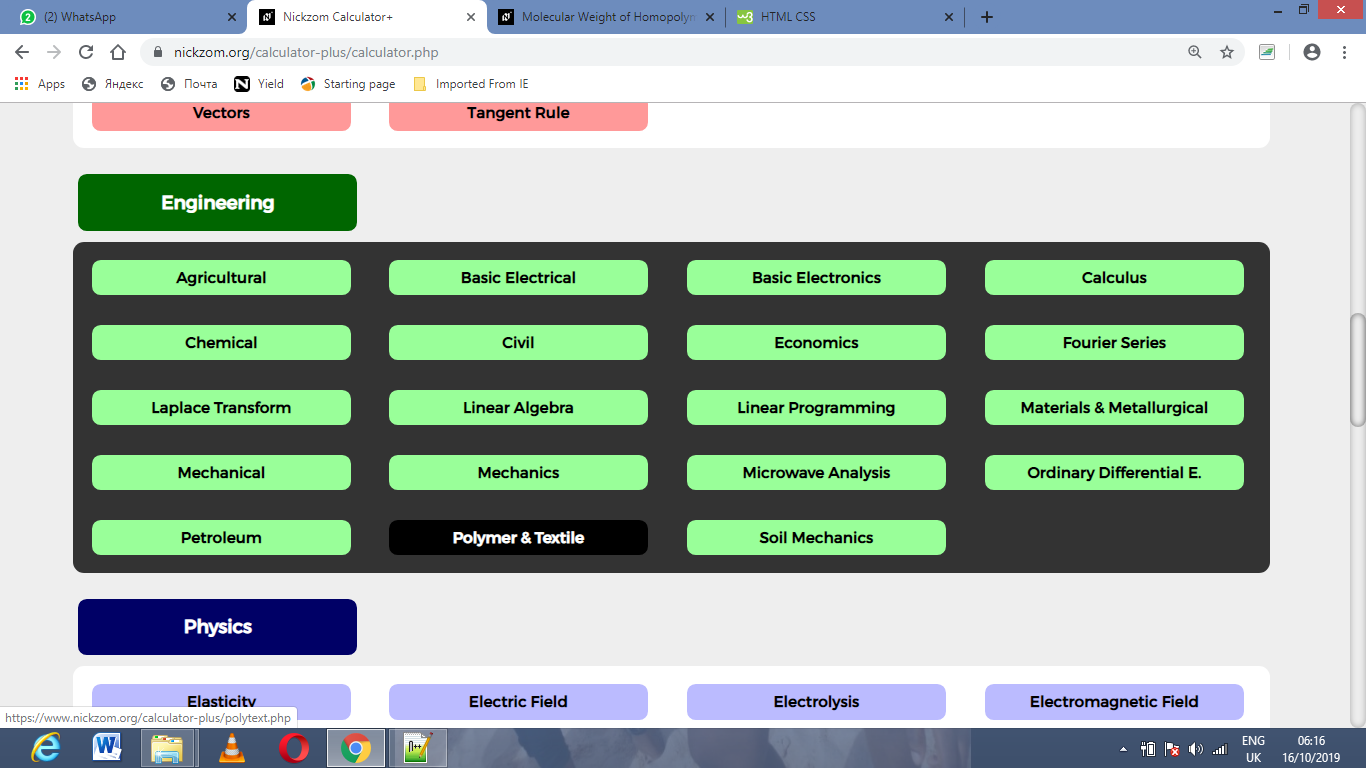Now, Click on Pressure Gradient under Polymer & Textile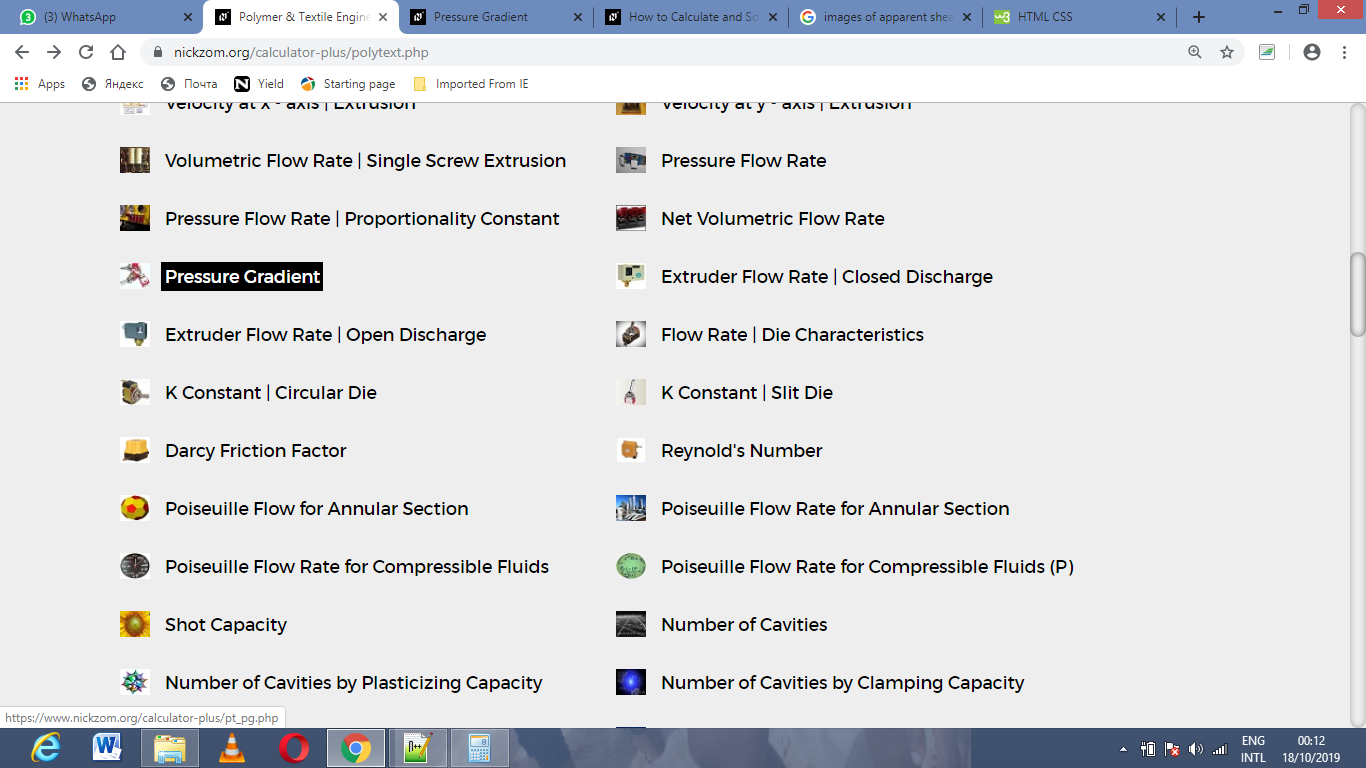The screenshot below displays the page or activity to enter your values, to get the answer for the pressure gradient according to the respective parameters which is the value (α), viscosity (μ), screw rotation speed (N) and proportionality constant that depends on screw geometry (B).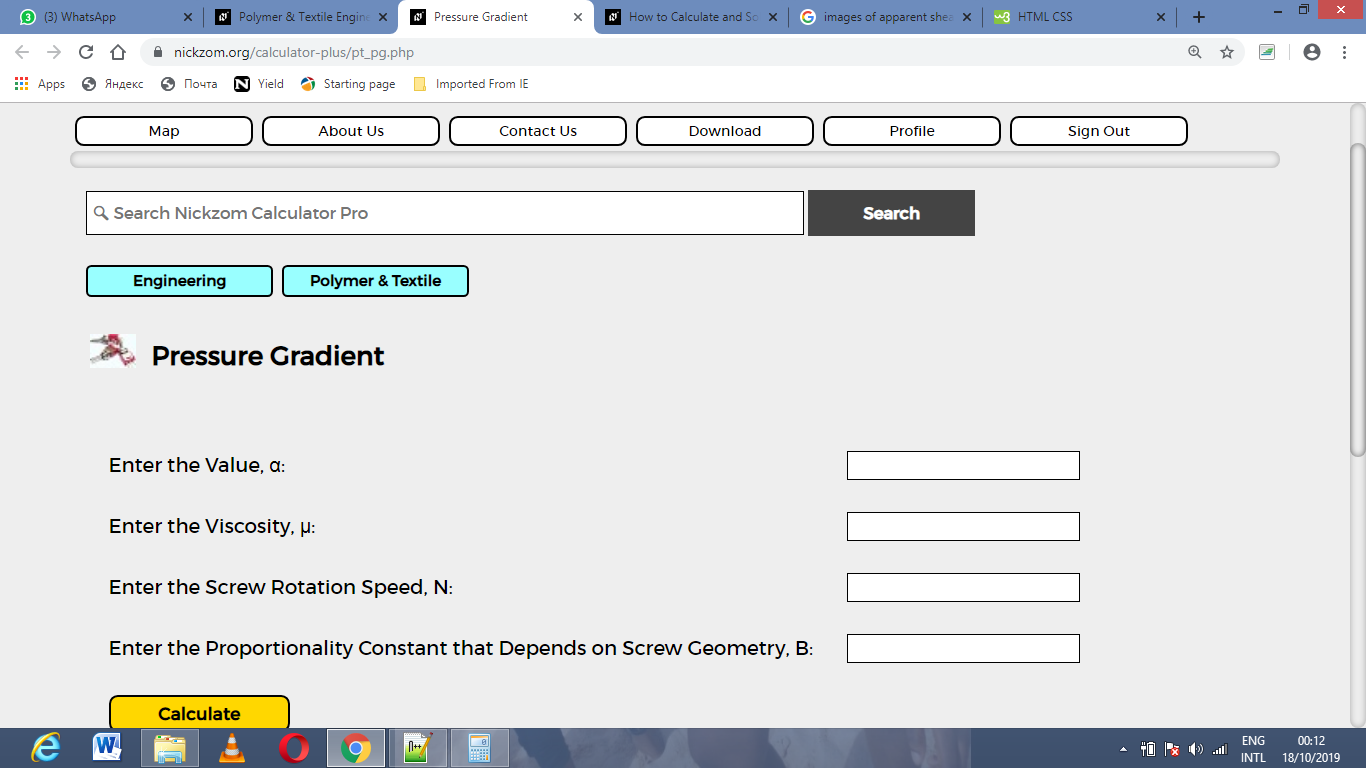Now, enter the values appropriately and accordingly for the parameters as required by the value (α) is 2, viscosity (μ) is 9, screw rotation speed (N) is 20 and proportionality constant that depends on screw geometry (B) is 24.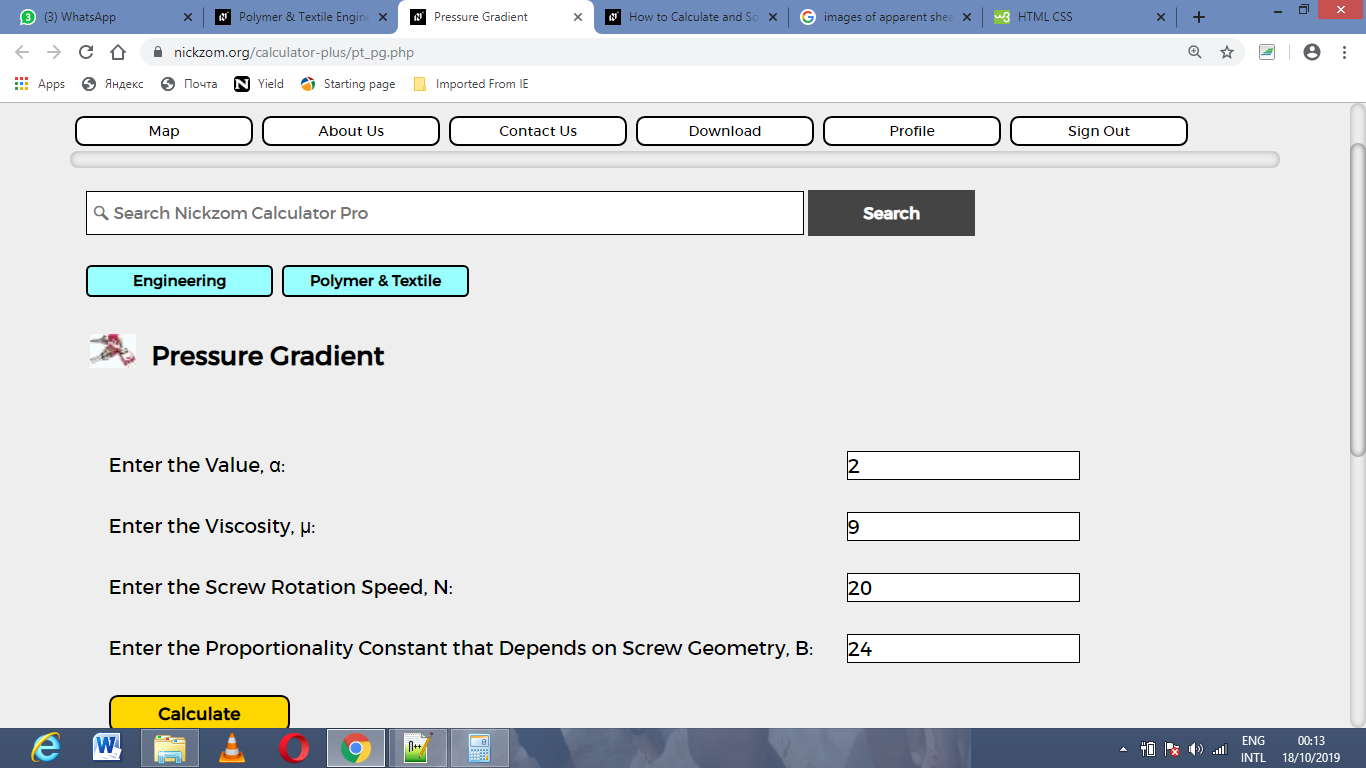Finally, Click on Calculate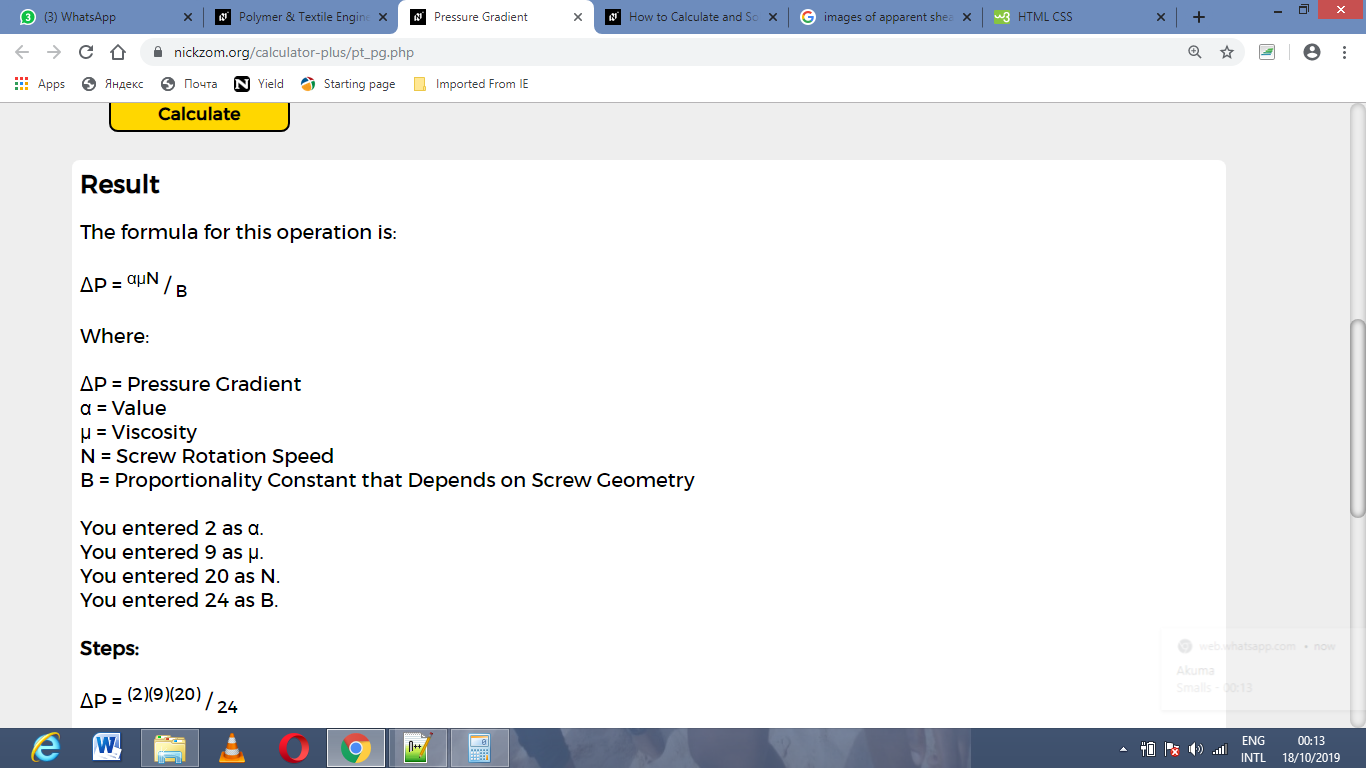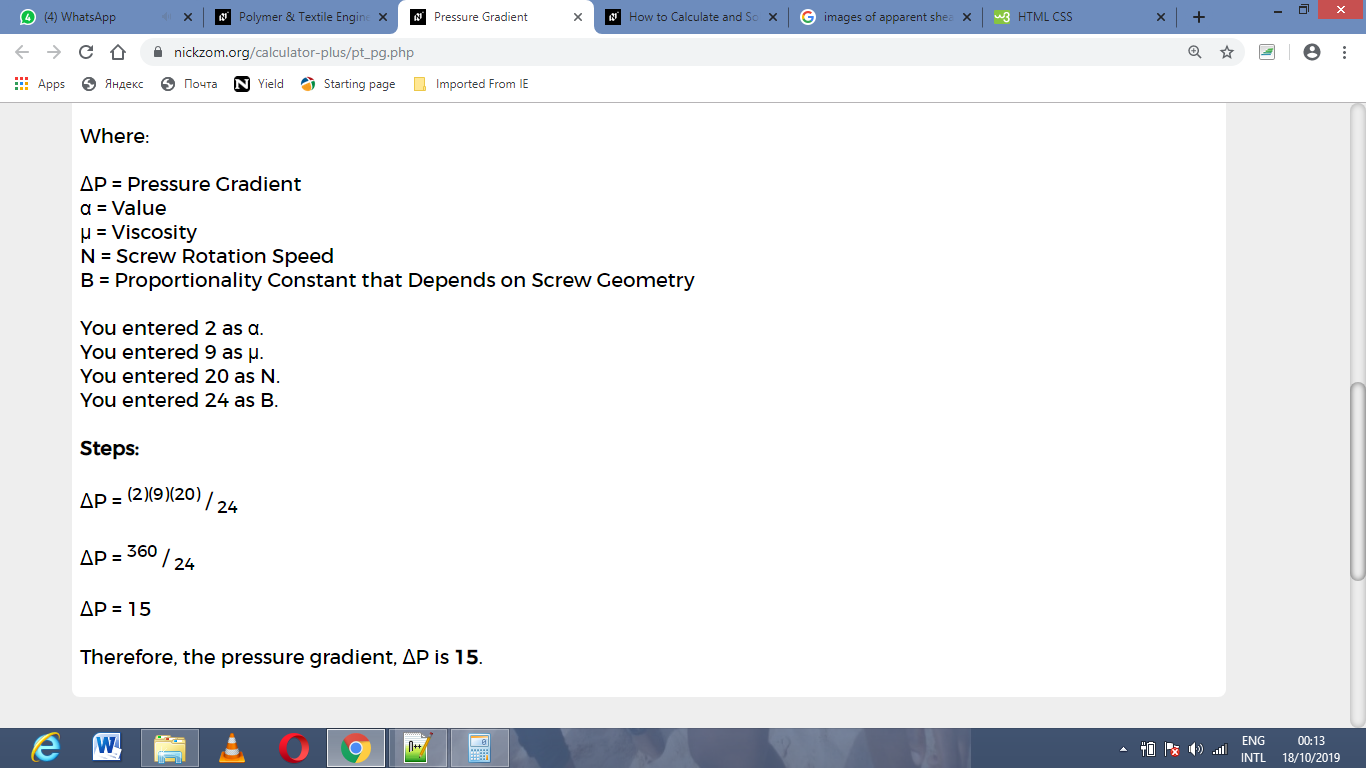As you can see from the screenshot above, Nickzom Calculator– The Calculator Encyclopedia solves for the pressure gradient and presents the formula, workings and steps too.